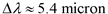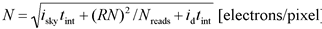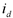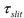Spitzer Documentation & Tools
IRS Instrument Handbook

### 2.10.5         Imaging Sensitivity to Point Sources

We use the same basic calculations presented above to estimate the sensitivity and S/N for PUI observations.  Table 2.4 summarizes the instrument sensitivity in the PUI mode.  The flux density is the peak-up target flux density at 16 microns (blue) or 22 microns (red).  The underlying procedure used to calculate the PUI sensitivities is presented for informative purposes here:

1.  The background photocurrent from sky and telescope is given by Table 2.4, where, in the case of PUI,.

2.  For faint sources, the total noise in electrons/pixel is the sum of the photocurrent shot noise, read noise, and dark current shot noise.  It is given by:Equation 2.13

whereis the dark current contribution, RN is the read noise in electrons, Nreads is the number of nondestructive samples up the ramp, and tint is the ramp time in seconds.

3.  The relationship between photocurrent per pixel and the incident flux density, SmJy, is given by Equation 2.4, except that the slit losses,, are replaced by the fraction of encircled energy in the assumed beam size, which, for PUI, is larger than the 4 pixels assumed in Equation 2.4.  For the nominal S/N calculations, we assumed a beam size equal to that of a circular aperture containing half the light of a point source.

4.  The S/N for a point source is then the ratio of SmJy to N.

Table 2.4 PUI 1σ sensitivity (microJy) for 6/14/30 second ramp times

 Filter LOW background MEDIUM background HIGH background Blue 115 / 75 / 50 145 / 95 / 65 190 / 120 / 80 Red 180 / 115 / 75 220 / 140 / 95 280 / 180 / 120